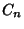## Catalan's Triangle

A triangle of numbers with entries given byfor, where each element is equal to the one above plus the one to the left. Furthermore, the sum of each row is equal to the last element of the next row and also equal to the Catalan Number.(Sloane's A009766).

See also Bell Triangle, Clark's Triangle, Euler's Triangle, Leibniz Harmonic Triangle, Number Triangle, Pascal's Triangle, Prime Triangle, Seidel-Entringer-Arnold Triangle

References

Sloane, N. J. A. Sequence A009766 in The On-Line Version of the Encyclopedia of Integer Sequences.'' http://www.research.att.com/~njas/sequences/eisonline.html.